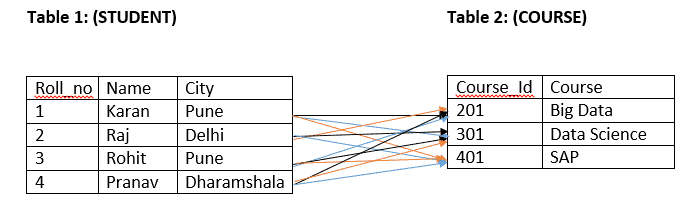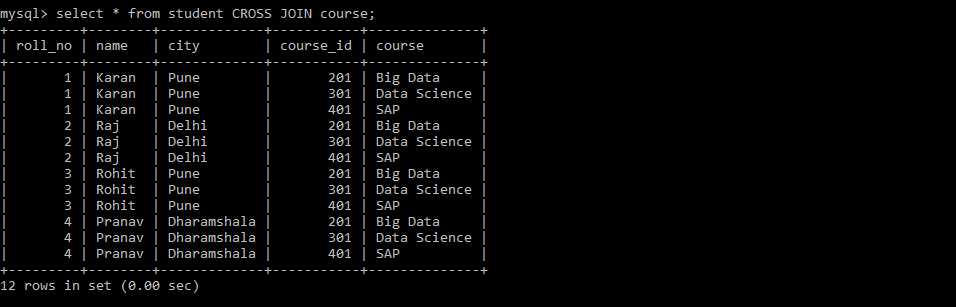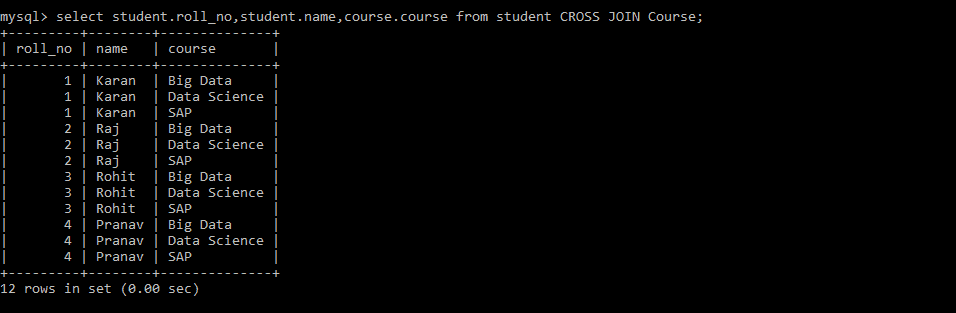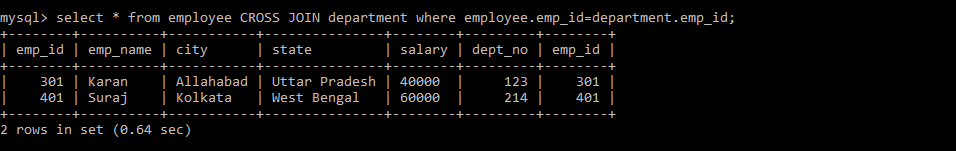# SQL CROSS JOIN: The Complete Guide

Cross join is also called a Cartesian product. Unlike an INNER JOIN or LEFT JOIN, the cross join does not establish the relationship between the joined tables.

Each row from the first table is combined with each row from the second table, known as Cartesian join or cross join.

## SQL CROSS JOIN

SQL CROSS JOIN clause combines rows from two or more tables based on a related column. SQL cross joins are used to join the table having no condition in which all the records of the first table come with all the records of the second table.

The CROSS JOIN joined every row from the first table (T1) with every row from the second table (T2).

In other words, the cross join returns the Cartesian product of rows from both tables. The number of rows in a Cartesian product is the product of the number of rows in each involved table.

Let’s say we have two tables, A and B.

The following statements perform the cross join and produce a cartesian product of the rows from the A and B tables.

```SELECT *
FROM A JOIN B;```
```SELECT *
FROM A
INNER JOIN B;```
```SELECT *
FROM A
CROSS JOIN B;```
```SELECT *
FROM A, B;```

Suppose the A table has N rows, and the B table has M rows, then the CROSS JOIN of these two tables will produce the result set that contains N x M rows.

Imagine that if you have a third table, C, with the K rows, the result of a CROSS JOIN clause of these three tables will contain N x M x K rows, which may be huge. You need to be very careful when using a CROSS JOIN clause.

You use an INNER JOIN and LEFT JOIN clauses more often than a CROSS JOIN clause. However, the CROSS JOIN clause is very useful in some use cases.

### Similarity Between INNER JOIN and CROSS JOIN

If a “WHERE” condition is used with CROSS JOIN, it will behave like an INNER JOIN.

#### NOTE:

If Where Condition is not used with CROSS JOIN, it will behave like a cartesian product.

### Syntax of Cross Join

`SELECT COLUMNS_NAME FROM TABLE_1 CROSS JOIN TABLE_2;`
1. In the above syntax, COLUMNS_NAME is the name of the columns which the user wants to join.
2. Table_1 and Table_2 are the names of the Tables.As you can see above, in Table 1, one row will be matched with all the rows in Table 2.

### SHOWING CROSS JOIN WITHOUT WHERE CONDITION

See the following query.

```Select * from STUDENT CROSS JOIN COURSE;
```
###### Output:You can also obtain this result without using CROSS JOIN Keyword.

See the following query.

`Select * from STUDENT, COURSE;`

#### Output### Other Examples:

Users can also select optional columns; not all columns are required for Cross Joining.

See the following query.

```Select student.roll_no,student.name,course.course
from student
CROSS JOIN Course;
```

See the output.See the table below.

##### Table_1: Employee
 Emp_id Emp_name City State Salary 101 Rohit Patna Bihar 30000 201 Shivam Jalandhar Punjab 20000 301 Karan Allahabad Uttar Pradesh 40000 401 Suraj Kolkata West Bengal 60000 501 Akash Vizag Andhra Pradesh 70000

#### Table_2: Department

 Dept_no Emp_id 123 301 214 401 125 505

See the following query.

```Select * from Employee
CROSS JOIN Department
where Employee.emp_id = Department.emp_id;
```

See the output.As mentioned above, the CROSS JOIN is similar to INNER JOIN if used with where condition.

Suppose there are m rows in Table 1 and n rows in Table 2

Then the total number of rows formed will be (m*n)

In the above example, there are four rows in Student and three rows in Course tables.

Therefore, the total number of rows formed is 12.

This is also known as cardinality number.

That’s it for this tutorial.

Categories SQL

This site uses Akismet to reduce spam. Learn how your comment data is processed.